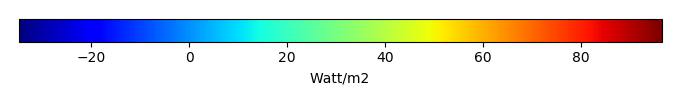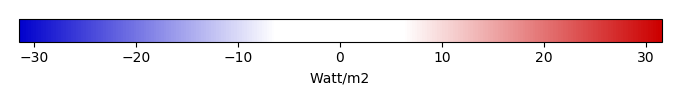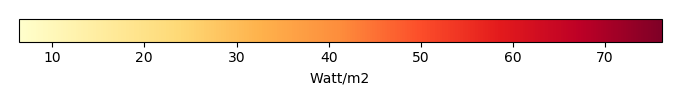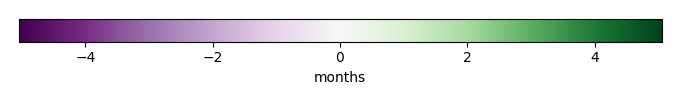# Mean State

Period Mean (original grids) [Watt/m2]
Model Period Mean (intersection) [Watt/m2]
Model Period Mean (complement) [Watt/m2]
Benchmark Period Mean (intersection) [Watt/m2]
Benchmark Period Mean (complement) [Watt/m2]
Bias [Watt/m2]
RMSE [Watt/m2]
Phase Shift [months]
Bias Score 
RMSE Score 
Seasonal Cycle Score 
Spatial Distribution Score 
Overall Score 
Benchmark [-] 44.1
CRUNCEPv7 [-] 51.8 51.1 64.4 44.2 12.4 7.31 18.6 1.46 0.41 0.24 0.79 0.76 0.49
GSWP3v1 [-] 45.4 45.0 52.0 44.2 12.4 1.09 14.3 1.87 0.48 0.27 0.70 0.95 0.53
WATCH [-] 36.0 35.3 39.8 44.3 12.5 -8.74 18.0 1.90 0.25 0.27 0.68 0.94 0.48
Period Mean (original grids) [Watt/m2]
Model Period Mean (intersection) [Watt/m2]
Model Period Mean (complement) [Watt/m2]
Benchmark Period Mean (intersection) [Watt/m2]
Benchmark Period Mean (complement) [Watt/m2]
Bias [Watt/m2]
RMSE [Watt/m2]
Phase Shift [months]
Bias Score 
RMSE Score 
Seasonal Cycle Score 
Spatial Distribution Score 
Overall Score 
Benchmark [-] 57.5
CRUNCEPv7 [-] 59.0 59.1 89.7 57.5 20.8 1.68 21.6 1.33 0.53 0.36 0.85 0.97 0.61
GSWP3v1 [-] 50.8 50.9 67.0 57.5 20.8 -6.70 20.0 0.772 0.45 0.43 0.94 0.84 0.62
WATCH [-] 38.2 38.5 63.7 57.7 20.6 -19.0 27.8 1.29 0.25 0.40 0.86 0.96 0.58
Period Mean (original grids) [Watt/m2]
Model Period Mean (intersection) [Watt/m2]
Model Period Mean (complement) [Watt/m2]
Benchmark Period Mean (intersection) [Watt/m2]
Benchmark Period Mean (complement) [Watt/m2]
Bias [Watt/m2]
RMSE [Watt/m2]
Phase Shift [months]
Bias Score 
RMSE Score 
Seasonal Cycle Score 
Spatial Distribution Score 
Overall Score 
Benchmark [-] 52.8
CRUNCEPv7 [-] 58.2 57.9 66.6 53.0 19.6 5.27 19.2 0.627 0.56 0.46 0.94 0.93 0.67
GSWP3v1 [-] 56.3 56.1 61.4 53.0 19.6 3.43 17.7 0.747 0.58 0.49 0.91 0.97 0.69
WATCH [-] 55.7 55.8 55.1 53.0 19.5 2.84 17.0 0.667 0.60 0.50 0.93 0.90 0.69
Period Mean (original grids) [Watt/m2]
Model Period Mean (intersection) [Watt/m2]
Model Period Mean (complement) [Watt/m2]
Benchmark Period Mean (intersection) [Watt/m2]
Benchmark Period Mean (complement) [Watt/m2]
Bias [Watt/m2]
RMSE [Watt/m2]
Phase Shift [months]
Bias Score 
RMSE Score 
Seasonal Cycle Score 
Spatial Distribution Score 
Overall Score 
Benchmark [-] 18.0
CRUNCEPv7 [-] -0.719 -0.431 -5.58 18.1 5.64 -18.8 25.0 0.610 0.46 0.55 0.95 0.52 0.61
GSWP3v1 [-] 15.6 16.0 7.19 18.1 5.64 -2.63 19.1 0.548 0.79 0.51 0.96 0.93 0.74
WATCH [-] 7.12 7.26 5.58 18.2 5.67 -10.9 20.1 0.494 0.63 0.54 0.96 0.86 0.71
Period Mean (original grids) [Watt/m2]
Model Period Mean (intersection) [Watt/m2]
Model Period Mean (complement) [Watt/m2]
Benchmark Period Mean (intersection) [Watt/m2]
Benchmark Period Mean (complement) [Watt/m2]
Bias [Watt/m2]
RMSE [Watt/m2]
Phase Shift [months]
Bias Score 
RMSE Score 
Seasonal Cycle Score 
Spatial Distribution Score 
Overall Score 
Benchmark [-] 22.1
CRUNCEPv7 [-] 29.9 28.6 36.4 22.8 10.8 8.44 22.3 1.78 0.13 0.14 0.73 0.42 0.31
GSWP3v1 [-] 32.3 31.3 36.9 22.8 10.8 10.5 16.4 1.34 0.31 0.20 0.80 0.87 0.47
WATCH [-] 27.4 27.1 28.8 22.8 10.8 5.04 14.1 1.78 0.35 0.16 0.73 0.94 0.47
Period Mean (original grids) [Watt/m2]
Model Period Mean (intersection) [Watt/m2]
Model Period Mean (complement) [Watt/m2]
Benchmark Period Mean (intersection) [Watt/m2]
Benchmark Period Mean (complement) [Watt/m2]
Bias [Watt/m2]
RMSE [Watt/m2]
Phase Shift [months]
Bias Score 
RMSE Score 
Seasonal Cycle Score 
Spatial Distribution Score 
Overall Score 
Benchmark [-] 27.0
CRUNCEPv7 [-] 10.9 10.9 15.3 27.1 8.81 -16.1 22.4 0.994 0.54 0.58 0.90 0.88 0.69
GSWP3v1 [-] 20.6 20.5 27.0 27.1 8.81 -6.35 15.9 0.553 0.73 0.60 0.95 0.93 0.76
WATCH [-] 14.9 14.8 22.0 27.1 8.84 -12.1 19.6 0.584 0.60 0.57 0.95 0.96 0.73
Period Mean (original grids) [Watt/m2]
Model Period Mean (intersection) [Watt/m2]
Model Period Mean (complement) [Watt/m2]
Benchmark Period Mean (intersection) [Watt/m2]
Benchmark Period Mean (complement) [Watt/m2]
Bias [Watt/m2]
RMSE [Watt/m2]
Phase Shift [months]
Bias Score 
RMSE Score 
Seasonal Cycle Score 
Spatial Distribution Score 
Overall Score 
Benchmark [-] 28.4
CRUNCEPv7 [-] 35.2 34.7 40.6 28.4 24.4 6.23 20.7 1.05 0.67 0.47 0.87 0.92 0.68
GSWP3v1 [-] 35.5 35.2 39.6 28.4 24.4 6.80 19.3 0.795 0.66 0.52 0.92 0.94 0.71
WATCH [-] 33.6 32.9 42.4 28.4 24.5 4.45 20.4 0.971 0.63 0.49 0.89 0.92 0.68
Period Mean (original grids) [Watt/m2]
Model Period Mean (intersection) [Watt/m2]
Model Period Mean (complement) [Watt/m2]
Benchmark Period Mean (intersection) [Watt/m2]
Benchmark Period Mean (complement) [Watt/m2]
Bias [Watt/m2]
RMSE [Watt/m2]
Phase Shift [months]
Bias Score 
RMSE Score 
Seasonal Cycle Score 
Spatial Distribution Score 
Overall Score 
Benchmark [-] 40.3
CRUNCEPv7 [-] 52.0 52.0 56.2 40.6 11.0 10.9 23.0 0.686 0.54 0.43 0.94 0.94 0.65
GSWP3v1 [-] 37.6 37.1 42.1 40.6 11.0 -3.33 20.5 0.882 0.50 0.46 0.91 0.88 0.64
WATCH [-] 35.4 35.3 38.4 40.7 11.1 -5.58 19.0 0.855 0.53 0.47 0.92 0.90 0.66
Period Mean (original grids) [Watt/m2]
Model Period Mean (intersection) [Watt/m2]
Model Period Mean (complement) [Watt/m2]
Benchmark Period Mean (intersection) [Watt/m2]
Benchmark Period Mean (complement) [Watt/m2]
Bias [Watt/m2]
RMSE [Watt/m2]
Phase Shift [months]
Bias Score 
RMSE Score 
Seasonal Cycle Score 
Spatial Distribution Score 
Overall Score 
Benchmark [-] 15.2
CRUNCEPv7 [-] -9.16 -8.77 -15.8 15.2 3.78 -24.2 28.4 0.787 0.38 0.57 0.94 0.20 0.53
GSWP3v1 [-] 4.37 4.77 -2.26 15.2 3.78 -10.7 22.1 0.816 0.64 0.49 0.93 0.85 0.68
WATCH [-] -0.608 -0.376 -3.94 15.3 3.83 -15.8 23.6 0.842 0.51 0.50 0.94 0.46 0.58
Period Mean (original grids) [Watt/m2]
Model Period Mean (intersection) [Watt/m2]
Model Period Mean (complement) [Watt/m2]
Benchmark Period Mean (intersection) [Watt/m2]
Benchmark Period Mean (complement) [Watt/m2]
Bias [Watt/m2]
RMSE [Watt/m2]
Phase Shift [months]
Bias Score 
RMSE Score 
Seasonal Cycle Score 
Spatial Distribution Score 
Overall Score 
Benchmark [-] 56.5
CRUNCEPv7 [-] 64.1 64.8 51.4 56.9 15.0 7.25 18.2 0.602 0.70 0.56 0.95 0.98 0.75
GSWP3v1 [-] 59.7 60.1 51.0 56.9 15.0 2.71 15.4 0.540 0.71 0.62 0.96 0.89 0.76
WATCH [-] 68.6 69.3 54.0 56.8 14.9 11.6 20.1 0.569 0.62 0.56 0.96 0.97 0.74
Period Mean (original grids) [Watt/m2]
Model Period Mean (intersection) [Watt/m2]
Model Period Mean (complement) [Watt/m2]
Benchmark Period Mean (intersection) [Watt/m2]
Benchmark Period Mean (complement) [Watt/m2]
Bias [Watt/m2]
RMSE [Watt/m2]
Phase Shift [months]
Bias Score 
RMSE Score 
Seasonal Cycle Score 
Spatial Distribution Score 
Overall Score 
Benchmark [-] 20.2
CRUNCEPv7 [-] 21.2 21.0 25.6 20.4 7.08 1.11 22.2 0.696 0.68 0.47 0.92 0.58 0.62
GSWP3v1 [-] 19.6 19.2 29.0 20.4 7.08 -0.160 16.0 0.832 0.72 0.60 0.92 0.82 0.73
WATCH [-] 14.1 13.9 19.4 20.4 7.11 -5.98 16.6 0.778 0.64 0.61 0.92 0.92 0.74
Period Mean (original grids) [Watt/m2]
Model Period Mean (intersection) [Watt/m2]
Model Period Mean (complement) [Watt/m2]
Benchmark Period Mean (intersection) [Watt/m2]
Benchmark Period Mean (complement) [Watt/m2]
Bias [Watt/m2]
RMSE [Watt/m2]
Phase Shift [months]
Bias Score 
RMSE Score 
Seasonal Cycle Score 
Spatial Distribution Score 
Overall Score 
Benchmark [-] 34.3
CRUNCEPv7 [-] 32.2 37.2 11.2 34.6 11.5 2.60 22.5 1.02 0.56 0.46 0.89 0.79 0.63
GSWP3v1 [-] 31.0 36.8 6.87 34.6 11.5 2.16 19.1 0.881 0.60 0.49 0.91 0.92 0.68
WATCH [-] 28.3 34.3 3.41 34.7 11.5 -0.436 20.1 0.948 0.54 0.48 0.90 0.90 0.66
Period Mean (original grids) [Watt/m2]
Model Period Mean (intersection) [Watt/m2]
Model Period Mean (complement) [Watt/m2]
Benchmark Period Mean (intersection) [Watt/m2]
Benchmark Period Mean (complement) [Watt/m2]
Bias [Watt/m2]
RMSE [Watt/m2]
Phase Shift [months]
Bias Score 
RMSE Score 
Seasonal Cycle Score 
Spatial Distribution Score 
Overall Score 
Benchmark [-] 22.7
CRUNCEPv7 [-] 56.4 58.3 54.6 22.8 18.3 36.2 44.1 1.34 0.51 0.45 0.93 0.80 0.63
GSWP3v1 [-] 45.5 48.2 43.0 22.8 18.3 26.2 36.6 1.26 0.51 0.49 0.94 0.78 0.64
WATCH [-] 35.7 37.3 34.2 22.8 18.4 15.1 32.7 1.45 0.43 0.48 0.92 0.68 0.60
Period Mean (original grids) [Watt/m2]
Model Period Mean (intersection) [Watt/m2]
Model Period Mean (complement) [Watt/m2]
Benchmark Period Mean (intersection) [Watt/m2]
Benchmark Period Mean (complement) [Watt/m2]
Bias [Watt/m2]
RMSE [Watt/m2]
Phase Shift [months]
Bias Score 
RMSE Score 
Seasonal Cycle Score 
Spatial Distribution Score 
Overall Score 
Benchmark [-] 17.0
CRUNCEPv7 [-] -1.36 -0.796 -10.9 17.0 4.51 -18.3 25.6 1.08 0.50 0.55 0.89 0.49 0.59
GSWP3v1 [-] 9.34 9.87 0.733 17.0 4.51 -7.63 21.0 0.966 0.71 0.51 0.91 0.88 0.70
WATCH [-] 5.43 5.71 -0.202 17.1 4.53 -11.4 22.1 1.01 0.63 0.53 0.90 0.76 0.67
Period Mean (original grids) [Watt/m2]
Model Period Mean (intersection) [Watt/m2]
Model Period Mean (complement) [Watt/m2]
Benchmark Period Mean (intersection) [Watt/m2]
Benchmark Period Mean (complement) [Watt/m2]
Bias [Watt/m2]
RMSE [Watt/m2]
Phase Shift [months]
Bias Score 
RMSE Score 
Seasonal Cycle Score 
Spatial Distribution Score 
Overall Score 
Benchmark [-] 47.7
CRUNCEPv7 [-] 54.1 53.3 62.2 48.2 15.9 5.59 24.9 0.650 0.46 0.52 0.95 0.68 0.63
GSWP3v1 [-] 50.2 49.8 55.9 48.2 15.9 1.46 21.3 0.676 0.51 0.53 0.93 0.88 0.68
WATCH [-] 50.8 50.4 53.4 48.4 16.0 1.51 18.4 0.608 0.58 0.55 0.95 0.94 0.72
Period Mean (original grids) [Watt/m2]
Model Period Mean (intersection) [Watt/m2]
Model Period Mean (complement) [Watt/m2]
Benchmark Period Mean (intersection) [Watt/m2]
Benchmark Period Mean (complement) [Watt/m2]
Bias [Watt/m2]
RMSE [Watt/m2]
Phase Shift [months]
Bias Score 
RMSE Score 
Seasonal Cycle Score 
Spatial Distribution Score 
Overall Score 
Benchmark [-] 28.6
CRUNCEPv7 [-] 31.5 31.5 33.6 28.7 12.1 3.05 17.9 1.89 0.63 0.40 0.70 0.83 0.59
GSWP3v1 [-] 30.5 30.4 32.8 28.7 12.1 1.84 14.4 0.637 0.73 0.47 0.93 0.98 0.72
WATCH [-] 35.1 35.2 33.8 28.7 12.1 6.65 16.5 0.707 0.62 0.47 0.93 0.91 0.68
Period Mean (original grids) [Watt/m2]
Model Period Mean (intersection) [Watt/m2]
Model Period Mean (complement) [Watt/m2]
Benchmark Period Mean (intersection) [Watt/m2]
Benchmark Period Mean (complement) [Watt/m2]
Bias [Watt/m2]
RMSE [Watt/m2]
Phase Shift [months]
Bias Score 
RMSE Score 
Seasonal Cycle Score 
Spatial Distribution Score 
Overall Score 
Benchmark [-] 26.7
CRUNCEPv7 [-] 17.4 17.4 17.9 26.9 8.98 -9.57 19.3 1.01 0.66 0.60 0.89 0.95 0.74
GSWP3v1 [-] 24.9 24.9 24.5 26.9 8.98 -1.95 16.2 0.693 0.78 0.59 0.94 0.97 0.78
WATCH [-] 24.8 24.9 23.2 26.9 8.98 -1.95 16.0 0.671 0.77 0.62 0.94 0.96 0.78
Period Mean (original grids) [Watt/m2]
Model Period Mean (intersection) [Watt/m2]
Model Period Mean (complement) [Watt/m2]
Benchmark Period Mean (intersection) [Watt/m2]
Benchmark Period Mean (complement) [Watt/m2]
Bias [Watt/m2]
RMSE [Watt/m2]
Phase Shift [months]
Bias Score 
RMSE Score 
Seasonal Cycle Score 
Spatial Distribution Score 
Overall Score 
Benchmark [-] 49.7
CRUNCEPv7 [-] 37.7 37.8 60.2 49.8 11.0 -11.9 19.2 0.577 0.64 0.66 0.95 0.92 0.76
GSWP3v1 [-] 44.9 44.6 66.6 49.8 11.0 -5.13 17.0 0.824 0.73 0.65 0.92 0.89 0.77
WATCH [-] 44.2 44.1 69.2 49.7 10.9 -5.44 16.5 0.653 0.73 0.67 0.94 0.91 0.79
Period Mean (original grids) [Watt/m2]
Model Period Mean (intersection) [Watt/m2]
Model Period Mean (complement) [Watt/m2]
Benchmark Period Mean (intersection) [Watt/m2]
Benchmark Period Mean (complement) [Watt/m2]
Bias [Watt/m2]
RMSE [Watt/m2]
Phase Shift [months]
Bias Score 
RMSE Score 
Seasonal Cycle Score 
Spatial Distribution Score 
Overall Score 
Benchmark [-] 30.2
CRUNCEPv7 [-] 36.5 36.4 50.4 30.3 13.0 6.57 16.1 1.45 0.33 0.19 0.81 0.70 0.45
GSWP3v1 [-] 30.7 30.7 45.7 30.3 13.0 0.943 12.6 1.42 0.43 0.28 0.81 0.70 0.50
WATCH [-] 35.8 35.9 31.6 30.3 12.9 5.52 14.6 1.34 0.35 0.23 0.82 0.75 0.48
Period Mean (original grids) [Watt/m2]
Model Period Mean (intersection) [Watt/m2]
Model Period Mean (complement) [Watt/m2]
Benchmark Period Mean (intersection) [Watt/m2]
Benchmark Period Mean (complement) [Watt/m2]
Bias [Watt/m2]
RMSE [Watt/m2]
Phase Shift [months]
Bias Score 
RMSE Score 
Seasonal Cycle Score 
Spatial Distribution Score 
Overall Score 
Benchmark [-] 40.3
CRUNCEPv7 [-] 46.8 47.0 43.6 40.4 9.36 6.69 16.7 0.967 0.51 0.42 0.90 0.78 0.61
GSWP3v1 [-] 42.8 43.1 40.0 40.4 9.36 2.70 14.4 0.875 0.61 0.43 0.91 0.88 0.65
WATCH [-] 40.3 40.7 38.4 40.3 9.38 0.367 14.8 1.04 0.57 0.43 0.88 0.88 0.64
Period Mean (original grids) [Watt/m2]
Model Period Mean (intersection) [Watt/m2]
Model Period Mean (complement) [Watt/m2]
Benchmark Period Mean (intersection) [Watt/m2]
Benchmark Period Mean (complement) [Watt/m2]
Bias [Watt/m2]
RMSE [Watt/m2]
Phase Shift [months]
Bias Score 
RMSE Score 
Seasonal Cycle Score 
Spatial Distribution Score 
Overall Score 
Benchmark [-] 41.5
CRUNCEPv7 [-] 56.9 56.4 61.4 42.0 6.56 13.2 26.8 0.781 0.61 0.55 0.93 0.94 0.72
GSWP3v1 [-] 62.7 62.0 68.8 42.0 6.56 18.5 33.8 0.746 0.50 0.51 0.94 0.83 0.66
WATCH [-] 65.6 65.2 70.6 42.0 6.58 22.0 30.9 0.588 0.54 0.53 0.95 0.93 0.70

# Temporally integrated period mean

BENCHMARK MEANMODEL MEANBIASBIAS SCORERMSERMSE SCOREBENCHMARK MAX MONTHMODEL MAX MONTHDIFFERENCE IN MAX MONTHSEASONAL CYCLE SCORESPATIAL TAYLOR DIAGRAMMODEL COLORS# Spatially integrated regional mean

MODEL COLORSREGIONAL MEANANNUAL CYCLEMONTHLY ANOMALYANNUAL CYCLE# All Models

BenchmarkCRUNCEPv7GSWP3v1WATCH# Data Information

creation_date: Tue Jul 28 15:54:11 PDT 2015

source_file: This product is generated from monthly Global Biosphere Amtospher Flux (GBAF) observations

title: derived GBAF in 0.5x0.5

Approach: I read this variable from data file, and then save the data in NetCDF format by each month and each year. Meanwhile, I also converted the unit from MJoule*m-2*d-1 to Watt/m2.

Temporal resolution: monthly

General information: This product was derived from Monthly estimates of global biosphere-atmosphere fluxes from Biogeochemistry group at Max Planck Institute in Jena, Germany.

Spatial resolution: 0.5x0.5 degree